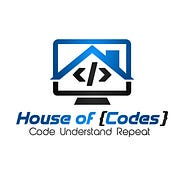# An eye-opener in Product

## Before Going Through the Code Here is a Beginner’s Guide to Java

Click on this link to get you to the landing page. It is completely free when you use kindle amazon. Take a look at it.

It is Completely free if you have Kindle Unlimited plan. It is worth the read if you want to learn java on your mobile and if you can’t take a paperback to each and every place you travel to. Let’s get to the Problem now

# Designer PDF Viewer

In this challenge, you will be given a list of letter heights in the alphabet and a string. Using the letter heights given, determine the area of the rectangle highlight in mm² assuming all letters are 1mm wide.

Input Format

The first line contains 26 space-separated integers describing the respective heights of each consecutive lowercase English letter, ASCII[a-z].
The second line contains a single word, consisting of lowercase English alphabetic letters.

Output Format

Print a single integer denoting the area in mm² of highlighted rectangle when the given word is selected. Do not print units of measure.

Code

static int designerPdfViewer(int[] h, String word) {
int max_h=h;
char words[]=word.toCharArray();
int i=0;
while(i<word.length())
{
if(h[words[i]-97]>max_h)
max_h=h[words[i]-97];
i++;
}
int ans=(i*max_h);
return ans;

}

Explanation

This problem asks us to find out the max height present in the given word. And to Calculate the value of area. Which is equal to the product of the,

total count of letters present in the word * the max height of the letter present in the word

Thus we have to find these two values. Then produce the output.

Algorithm

• Convert the given string into an array of Characters. words[].
• Initialize the value of max to be the first element of the array h. which holds the value of the heights of the all the letters
• Traverse through the array words[], If you find the count of that letter is higher than max.

Change the value of max to the current value

• At the end of the traversal, we would have find out the highest length of the letter present in the word.( the max height of the letter present in the word)
• Now, the value of i at the end of the traversal would be equal to the length of the word.( total count of letters present in the word)
• We have find out the individual elements present in the total product.
• We have to multiply these two values. And Print the answer.

Working of the code

## 1 3 1 3 1 4 1 3 2 5 5 5 5 5 5 5 5 5 5 5 5 5 5 5 5 7zaba

max_height=1;

i=0;

Iteration 1:

h[‘z’-97]=>h=>7;if(h>max_height);(7>1);true

max_height=7;i=0;

Iteration 2:

h[‘a’-97]=>h=>1;if(h>max_height);(1>7);false

max_height=7;i=1;

Iteration 3:

h[‘b’-97]=>h=>3;if(h>max_height);(3>7);false

max_height=7;i=2;

Iteration 4:

h[‘a’-97]=>h=>1;if(h>max_height);(1>7);false

max_height=7;i=3;

End of traversal

max_height=7;i=4;

ans=(max_height*i);

ans=(7*4)=28mm²;

Conclusion

No Single Solution exists to a problem.Work on the Code. Share your Thoughts with me. These problems are from HackerRank.